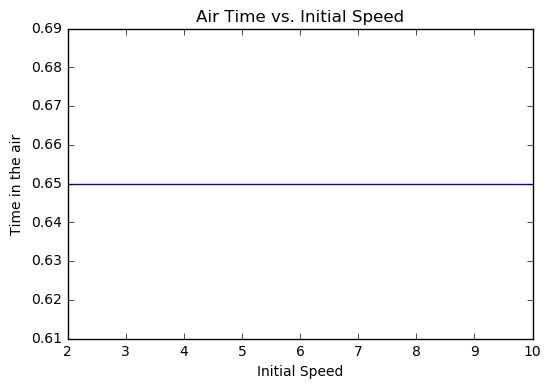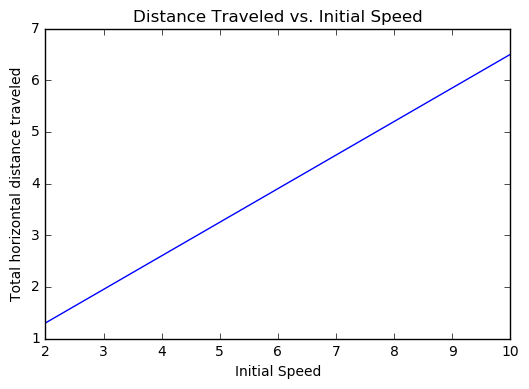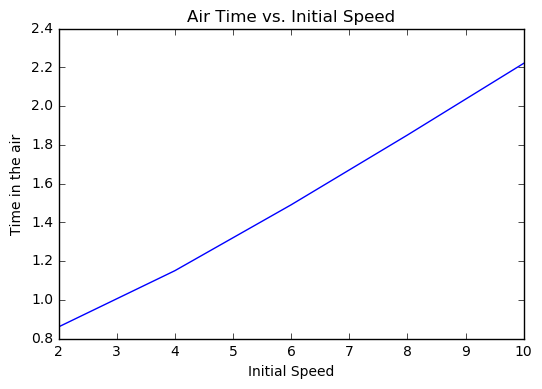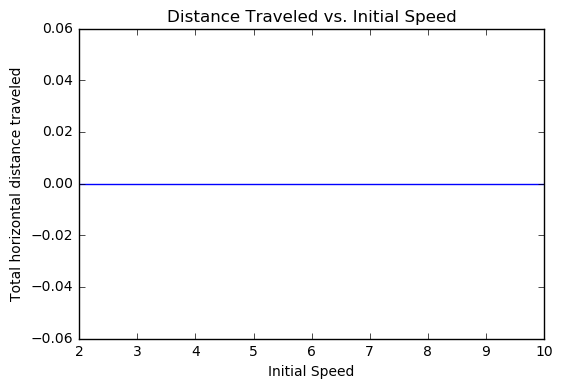CoCalc Shared FilesPICUP / Projectile Motion Computational Model and Experiment / ball_launch_lab_activity.html
Author: Todd Zimmerman
Views : 7
Description: Jupyter html version of PICUP/Projectile Motion Computational Model and Experiment/ball_launch_lab_activity.ipynb
ball_launch_lab_activity
In :
#Exercise 1
from vpython import *
from math import *
import matplotlib.pyplot as plt
%matplotlib inline

scene=canvas(title='Ball Spring Launcher')   #Sets title for graphics window
scene.range=5    #Determines how wide graphics window is (sorta)
scene.center=vector(0,0,0)

dt = 0.01    #dt is the time step 'Delta t'
t=0   #Set initial time to zero
g = vector(0,-9.8,0)
h = 2   #initial height of ball
v_init=10

ball = sphere(pos=vector(0,h,0), radius=.2, color=color.red,make_trail=True)   #Create sphere that will appear on screen
ground = box(pos=vector(0,0,0),color=color.green,size=vector(10,.1,5))  #Create green "grass" to give a reference frame
table = box(pos=vector(-0.5,h/2,0), color=color.blue, size=vector(1,h,1))  #Create a "table" for reference
ball.m = 1   #Mass of ball
ball.v = vector(v_init,0,0)    #Initial velocity vector of ball

scene.waitfor("click")   #Don't go onto the next step until you have clicked on the screen
while ball.pos.y>0:  #Stop when ball hits floor
rate(40)   #Determines how quickly program runs (roughly 30 frames per second)
ball.pos = ball.pos + ball.v*dt  #Update position of ball
ball.v = ball.v + g*dt  #Update velocity of ball
t+=dt   #Calculate total time elapsed

print("Time elapsed = ", t)
print("Horizontal distance traveled = ", ball.pos.x)

('Time elapsed = ', 0.6500000000000004)
('Horizontal distance traveled = ', 6.499999999999993)

In :
#Exercise 2
import matplotlib.pyplot as plt
%matplotlib inline

init_velocity = [ 2, 4, 6, 8, 10]
time_in_air = [0.65, 0.65, 0.65, 0.65, 0.65  ]
distance_traveled = [1.3, 2.6, 3.9, 5.2,6.5  ]

plt.plot(init_velocity, time_in_air)
plt.title('Air Time vs. Initial Speed')
plt.xlabel('Initial Speed')
plt.ylabel('Time in the air')
plt.show()

plt.plot(init_velocity, distance_traveled)
plt.title('Distance Traveled vs. Initial Speed')
plt.xlabel('Initial Speed')
plt.ylabel('Total horizontal distance traveled')
plt.show()In :
#Exercise 3
from vpython import *
from math import *

scene=canvas(title='Ball Spring Launcher')   #Sets title for graphics window
scene.range=5    #Determines how wide graphics window is (sorta)
scene.center=vector(0,0,0)

dt = 0.01    #dt is the time step 'Delta t'
t=0   #Set initial time to zero
g = vector(0,-9.8,0)
h = 2   #initial height of ball
theta=pi/4
v_init=5
v=vector(v_init*cos(theta),v_init*sin(theta),0)

ball = sphere(pos=vector(0,h,0), radius=.2, color=color.red, make_trail=True)   #Create sphere that will appear on screen
ground = box(pos=vector(0,0,0),color=color.green,size=vector(10,.1,5))  #Create green "grass" to give a reference frame
table = box(pos=vector(-0.5,h/2,0), color=color.blue, size=vector(1,h,1))  #Create a "table" for reference
ball.m = 1   #Mass of ball
ball.v = v    #Initial velocity vector of ball

scene.waitfor("click")   #You must click on the image before it will start moving
while ball.pos.y>0+ball.radius:  #Stop when ball hits floor
rate(40)   #Determines how quickly program runs (roughly 30 frames per second)
ball.pos = ball.pos + ball.v*dt  #Update position of ball
ball.v = ball.v + g*dt  #Update momentum of ball
t+=dt   #Calculate total time elapsed

print("Time elapsed = ", t)
print("Horizontal distance traveled = ", ball.pos.x)

('Time elapsed = ', 1.0800000000000007)
('Horizontal distance traveled = ', 3.8183766184073518)

In :
#Exercise 4
import matplotlib.pyplot as plt
%matplotlib inline

init_velocity = [ 2, 4, 6, 8, 10]
time_in_air = [0.86, 1.15, 1.49, 1.85, 2.22  ]
distance_traveled = [0, 0, 0, 0,0  ]

plt.plot(init_velocity, time_in_air)
plt.title('Air Time vs. Initial Speed')
plt.xlabel('Initial Speed')
plt.ylabel('Time in the air')
plt.show()

plt.plot(init_velocity, distance_traveled)
plt.title('Distance Traveled vs. Initial Speed')
plt.xlabel('Initial Speed')
plt.ylabel('Total horizontal distance traveled')
plt.show()In [ ]: